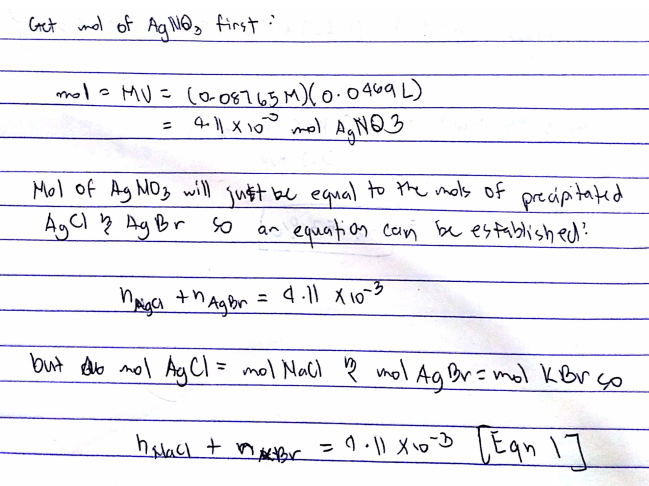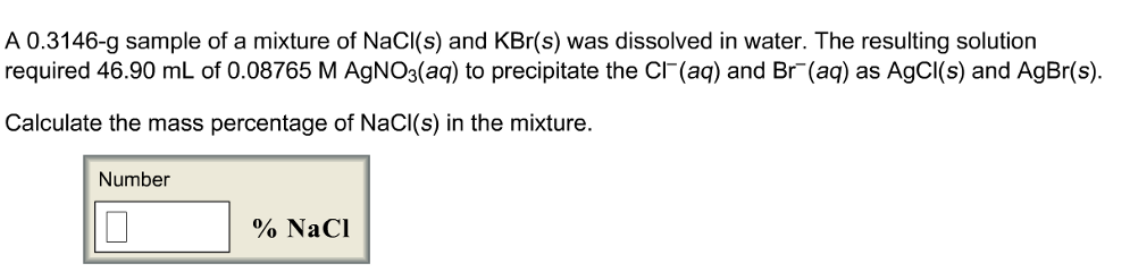# Problem: A 0.3146-g sample of a mixture of NaCI(s) and KBr(s) was dissolved in water. The resulting solution required 46.90 mL of 0.08765 M AgNO3(aq) to precipitate the Cl^-(aq) and Br^-(aq) as AgCI(s) and AgBr(s). Calculate the mass percentage of NaCI(s) in the mixture.

###### FREE Expert Solution98% (471 ratings)###### Problem Details

A 0.3146-g sample of a mixture of NaCI(s) and KBr(s) was dissolved in water. The resulting solution required 46.90 mL of 0.08765 M AgNO3(aq) to precipitate the Cl^-(aq) and Br^-(aq) as AgCI(s) and AgBr(s). Calculate the mass percentage of NaCI(s) in the mixture.